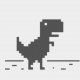# Slopes for 3D model## Recommended PostsHello,

I have a file attached. It's the design for a yard.

I want to annotate the slope of the planes. I could draw a line and annotate the slope of that line as well.

Is there a lisp program able to calculate the slope for planes/lines drawn in 3D?

##### Share on other sites```(defun c:?? (/ *error* os a d p1 p2 p % lst)
;hp 14.07.2020
(defun *error* (msg)
(ai_sysvar nil)
(terpri)
(princ msg)
)

(ai_sysvar '(( "OSNAPZ" . 0) ( "OSMODE" . 522)))
(while (and (setq p1 (getpoint "\nSpecify point.. "))
(setq p2 (getpoint p1 "\nNext point.. "))
)
(setq lst (list p1 p2)
lst (mapcar '(lambda (x) (list (car x) (cadr x))) lst)
a (apply 'angle lst)
d (apply 'distance lst)
)
(not (zerop d))
(setq % (/ (caddr (mapcar '- p2 p1)) d))
(if (minusp %)
(mapcar 'set '(p1 p2) (reverse lst))
lst
)
(setq p (trans (apply 'mapcar (cons '(lambda (a b) (* 0.5 (+ a b))) lst))1 0))
(entmakex (list '(0 . "TEXT") (cons 1 (strcat (if (minusp %) "\U+2192 " "\U+2190 ")))
(cons 40 (* d 0.1)) (cons 10 p) (cons 11 p) (cons 50 a) '(72 . 0) '(73 . 2)
)
)
)
(ai_sysvar nil)
(princ)
)```

p/s: text style use TTF  (eg: Arial, calibri etc..) not SHX

##### Share on other sites24 minutes ago, hanhphuc said:
```
(defun c:?? (/ *error* os a d p1 p2 p % lst)
;hp 14.07.2020
(defun *error* (msg)
(ai_sysvar nil)
(terpri)
(princ msg)
)

(ai_sysvar '(( "OSNAPZ" . 0) ( "OSMODE" . 522)))
(while (and (setq p1 (getpoint "\nSpecify point.. "))
(setq p2 (getpoint p1 "\nNext point.. "))
)
(setq lst (list p1 p2)
lst (mapcar '(lambda (x) (list (car x) (cadr x))) lst)
a (apply 'angle lst)
d (apply 'distance lst)
)
(not (zerop d))
(setq % (/ (caddr (mapcar '- p2 p1)) d))
(if (minusp %)
(mapcar 'set '(p1 p2) (reverse lst))
lst
)
(setq p (trans (apply 'mapcar (cons '(lambda (a b) (* 0.5 (+ a b))) lst))1 0))
(entmakex (list '(0 . "TEXT") (cons 1 (strcat (if (minusp %) "\U+2192 " "\U+2190 ")))
(cons 40 (* d 0.1)) (cons 10 p) (cons 11 p) (cons 50 a) '(72 . 0) '(73 . 2)
)
)
)
(ai_sysvar nil)
(princ)
)```

p/s: text style use TTF  (eg: Arial, calibri etc..) not SHX

this gives me a question mark every time....

##### Share on other sites12 minutes ago, Lippens Infra said:

this gives me a question mark every time....

missing font type

36 minutes ago, hanhphuc said:

p/s: text style use TTF  (eg: Arial, Calibri etc..) not SHX

check your Text Style as mentioned

##### Share on other sites2 minutes ago, hanhphuc said:

missing font type

check your Text Style as mentioned

now I only have an arrow, my bad for the text style. I was not attentive.

##### Share on other sitesI've seen a similar question and this code by LRM solved my problem! thanks for that!

```;; Determine the maximum slope of a 3dface.
;; 7/13/2020
(defun c:FaceSlope (/ ss en edata p1 p2 p3 v1 sv a slope midpt s endpt)
(setq oldsnap (getvar "osmode"))
(setvar "osmode" 0)
(princ "\nPlease select 3DFACE and press ENTER.")
(setq	ss    (ssget)
en    (ssname ss 0)
edata (entget en)
)
(setvar "cmdecho" 0)
(if (= (cdr (assoc 0 edata)) "3DFACE")
(progn
(setq p1 (cdr (assoc 10 edata))	;set p1, p2, p3 to the three vertices of the 3DFACE
p2 (cdr (assoc 11 edata))
p3 (cdr (assoc 12 edata))
)
(if (or (equal p1 p2 0.0001)
(equal p1 p3 0.0001)
(equal p3 p2 0.0001)
)
(princ "\nThe first 3 vertices of the face are not unique.")
(progn
(setq normal (cross (mapcar '- p2 p1) (mapcar '- p3 p1)))
(setq v1 (cross '(0.0 0.0 1.0) normal))
(setq sv (cross v1 normal))
(setq a (distance '(0 0 0) sv))
(setq sv (mapcar '/ sv (list a a a)))
(setq a (expt (+ (expt (car sv) 2) (expt (cadr sv) 2)) 0.5))
;; check if a = 0
(if (< (abs a) 0.00001)
(setq slope "Vertical")
(setq slope (/ (caddr sv) a))
)
(princ "\nThe slope is: ")
(princ slope)
(princ "\nThe slope vector is: ")
(princ sv)

(setq midpt (mapcar '/ (mapcar '+ p1 p2 p3) '(3.0 3.0 3.0)))
(setq
s (/ (+ (distance p1 p2) (distance p2 p3) (distance p1 p3)) 3.0)
)
(setq endpt (mapcar '+ midpt (mapcar '* sv (list s s s))))
(command "_line" midpt endpt "")
;; draw line showing maximum slope

(setq slope (LM:roundto slope 3))
(command "text" midpt "" "" slope "")
)				; end if false, no duplicates
)					;end true, is face
)					; end if duplicate
(princ "\nSelected object must be a face.")
)					; end if face
(setvar "osmode" oldsnap)
(setvar "cmdecho" 1)
(princ)
)
;;; Compute the cross product of 2 vectors a and b
(defun cross (a b / crs)
(setq	crs (list
(- (* (nth 1 a) (nth 2 b))```

## Join the conversation

You can post now and register later. If you have an account, sign in now to post with your account.
Note: Your post will require moderator approval before it will be visible.Unfortunately, your content contains terms that we do not allow. Please edit your content to remove the highlighted words below.

×   Pasted as rich text.   Restore formatting

Only 75 emoji are allowed.